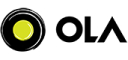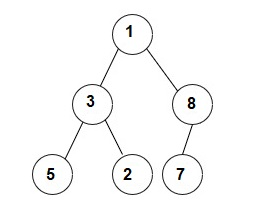New update is available. Click here to update.
Last Updated: 23 Nov, 2020

# Size of Largest BST in Binary Tree

Easy+3 more companies

## Problem statement#### A binary search tree (BST) is a binary tree data structure which has the following properties.

``````• The left subtree of a node contains only nodes with data less than the node’s data.
• The right subtree of a node contains only nodes with data greater than the node’s data.
• Both the left and right subtrees must also be binary search trees.
``````

##### Example:
``````Given binary tree:
````````````In the given binary tree, subtree rooted at 2 is a BST and its size is 3.
``````
##### Input Format
``````The first line contains elements in the level order form. The input consists of values of nodes separated by a single space in a single line.

In case a node is null, we take -1 on its place.
``````
##### Example :
``````The input for the tree is depicted in the below image:
````````````1 3 8 5 2 7 -1 -1 -1 -1 -1 -1 -1
``````
##### Explanation :
``````Level 1 :
The root node of the tree is 1

Level 2 :
Left child of 1 = 3
Right child of 1 = 8

Level 3 :
Left child of 3 = 5
Right child of 3 = 2
Left child of 8 =7
Right child of 8 =  null (-1)

Level 4 :
Left child of 5 = null (-1)
Right child of 5 = null (-1)
Left child of 2 = null (-1)
Right child of 2 = null (-1)
Left child of 7 = null (-1)
Right child of 7 = null (-1)

1
3 8
5 2 7 -1
-1 -1 -1 -1 -1 -1
``````
##### Note :
``````1. The first not-null node(of the previous level) is treated as the parent of the first two nodes of the current level. The second not-null node (of the previous level) is treated as the parent node for the next two nodes of the current level and so on.

2. The input ends when all nodes at the last level are null(-1).

3. The above format was just to provide clarity on how the input is formed for a given tree. The sequence will be put together in a single line separated by a single space. Hence, for the above-depicted tree, the input will be given as:

1 3 8 5 2 7 -1 -1 -1 -1 -1 -1 -1
``````
##### Output Format :
``````The output contains an integer denoting the largest size of the subtree of the binary tree which is also a BST.
``````
##### Note:
``````You do not need to print anything; it has already been taken care of. Just implement the given function.
``````## Approaches

### 01 ApproachWe can make every node of the binary tree as the root node BST and check whether it is a BST or not.

The algorithm will be-

• We will start from the root of the binary tree and do the inorder traversal using recursion.
• For the current node ‘ROOT', we will do the following-
• If it is the root of a valid BST, we return its size.
• Else, we will find the largest BST in left and right subtrees.